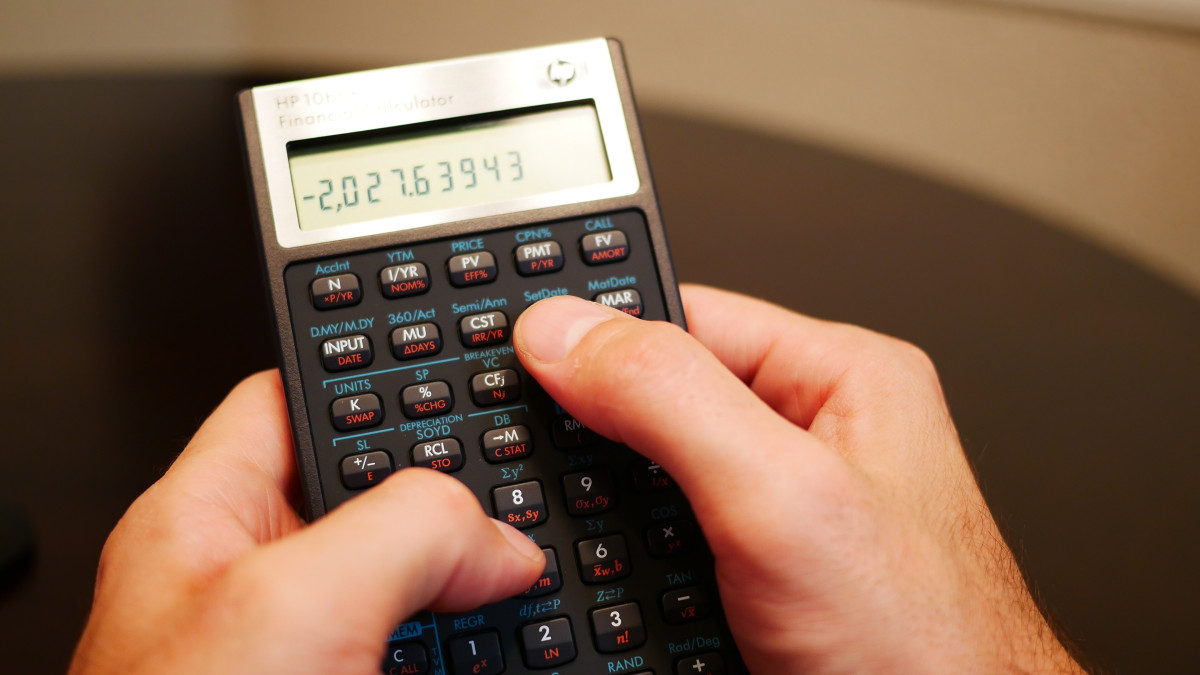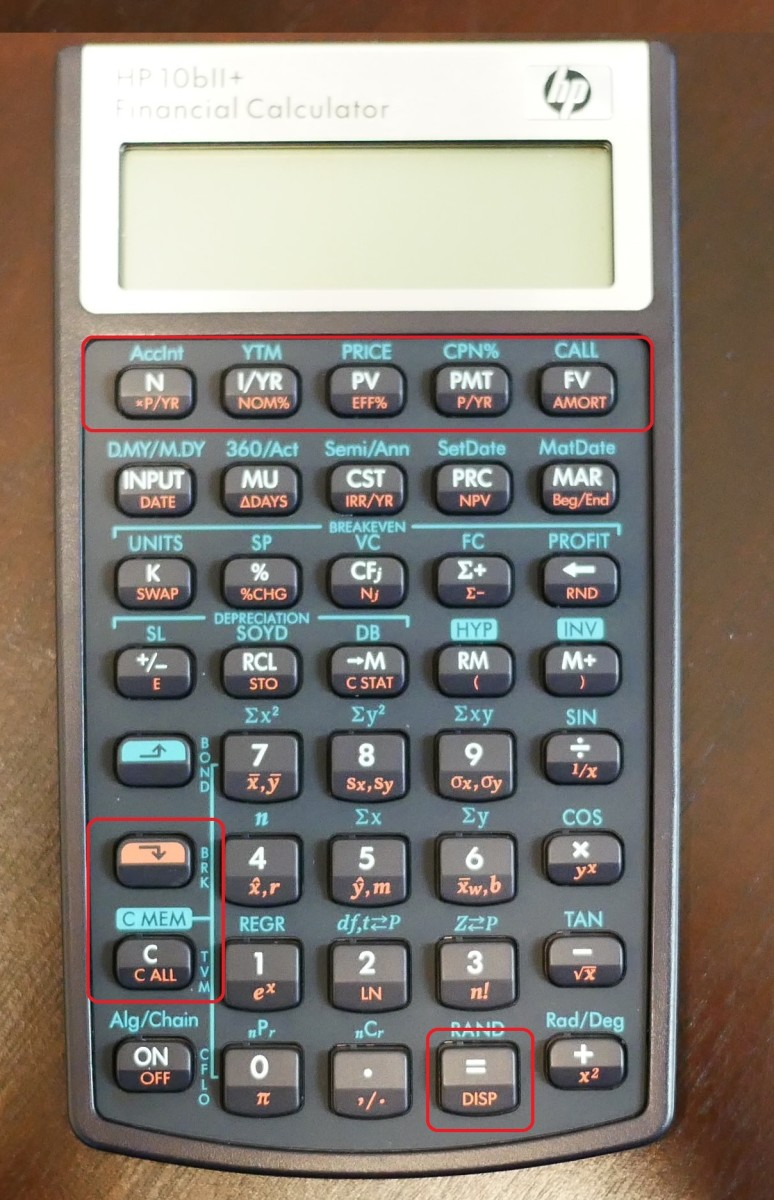# How to Answer Amortization Problems With an HP 10bII+ Calculator

Joshua earned an MBA from USF and he writes mostly about software and technology.

## What Is Amortization?

Amortization occurs when a payment plan to reduce debt by a stated date
has a portion of principal and interest in the payment.

## HP 10bii CalculatorThe HP 10bii+ is an upgrade to the HP 10bii. The upgrade was HP's idea to better utilize the keyboard of the calculator by adding functionality. New functions that this calculator has are probability distribution calculations, depreciation, & bonds.Created by Joshua Crowder

Solving an amortization problem can be easy if you have all of the necessary data. To solve this type of problem by hand, you normally have to organize your data into a table. This can become very time-consuming for a problem that is amortized over the course of 10 years! As long as you have enough information to calculate the payment, you will be able to reference all relevant data with the HP 10bII+ calculator. In order to get similar answers when you follow along, make sure that your calculator is set up for annual interest per year.

## Buttons UsedThe 5 standard variables located in the top row of keys that are used for the time value of money calculations are N (Number of payments), I/YR (Annual interest rate), PV (Present value), PMT (periodic payment amount), FV (Future Value).Created by Joshua Crowder

## Sample Problem

Let’s say you borrow \$100,000 and make annual payments for 3 years. If the interest rate is 7%, how much interest do you pay in year 2?

The first thing we want to do is clear the calculator. Press the orange shift key and the button labeled "C ALL" in orange text ("C" in white text ) consecutively. The calculator should display "1 P_Yr."

## Enter Your Data

1. Type 3, then press the "N" button (There are three payment periods)
2. Type 7, then press the "I/YR" button (Do not input the symbol %)
3. Type 100,000, then press the "PV" button (This is positive because you received cash)
4. Type 0, then press the "FV" button (If you cleared your calculator when you started you can skip this step)

After pressing the N, I/YR, PV, and FV buttons, the inputted data will be saved in the calculator.

## Display the Data

To display the fixed payment, press the "PMT" button. Your calculator should display a cash outflow of -38,105.17. This is the annual payment that you would have to pay to borrow this money. Now that we know the fixed payment, let's look at the other details. Press the orange shift button, then press the "AMORT" button. The calculator will display 1-1 letting you know it is displaying data for the first period. To cycle through all of your period 1 data, press the equal button. See the results for period 1 below:

Principle -31,105.17

Interest -7,000.00

Balance 68,894.83

Now that you have reviewed period 1 data, let's see what period 2 data holds. Press the orange shift button and press the "AMORT" button. The calculator will display 2-2 to let you know that you are in period 2. Now use the equal button to review the data. See the results for period 2 below:

Scroll to Continue

## Read More From Toughnickel

Principle -33.282.53

Interest -4,822.64

Balance 35,612.31

To answer the problem above, interest paid in year 2 would be \$4,822.64. Remember that your answer should remain a positive number even though your calculator displays the number as a negative cash flow.

Below is an amortization table/schedule created in MS Excel. This table took me about eight minutes to create in excel. With the calculator, I was able to complete the table in about two minutes.

## Monthly Payment Example

Annual payments were made in our example above. Let's try the same problem but change annual payments to monthly payments. In this situation, you must find the number of periods and the periodic rate. This can be done by completing the calculation right before entering data to the "N" or "I/YR" buttons.

If the original example problem above stated that monthly payments would be made, then we would have to multiply 3 times 12 to give us 36 total payments. So immediately after we make this calculation we can press the "N" button. Now that we are making 36 payments over the course of 3 years, our payment should be significantly smaller. Next, we have to figure out the periodic interest rate. This can be found by dividing the interest by the number of periods per year. When entering interest, type 7 / 12 = and then press the "I/YR" button. See below for data entry instructions.

1. Type 3 x 12 =, then press the "N" button
2. Type 7 / 12 =, then press the "I/YR" button
3. Type 100,000, then press the "PV" button
4. Type 0, then press the "FV" button

When you press the "PMT" button to retrieve the value of the payment the result should be -3,087.71 and your interest payment in period 2 would be \$568.72.

## Quarterly Payment Example

If the original problem above stated that quarterly payments would be made, then we would have to multiply 3 times 4 to give us 12 total payments. The periodic interest rate can be found by dividing the interest by the number of periods per year. When entering interest, type 7 / 4 = and then press the "I/YR" button. See below for data entry instructions.

1. Type 3 x 4 =, then press the "N" button
2. Type 7 / 4 =, then press the "I/YR" button
3. Type 100,000, then press the "PV" button
4. Type 0, then press the "FV" button

When you press the "PMT" button to retrieve the value of the payment the result should be -9,311.38 and your interest payment in period 2 would be \$1,617.68.

Cornell Law School. (n.d.). Amortization. Retrieved January 14, 2020, from https://www.law.cornell.edu/wex/amortization.

This content is accurate and true to the best of the author’s knowledge and is not meant to substitute for formal and individualized advice from a qualified professional.

© 2018 Joshua Crowder